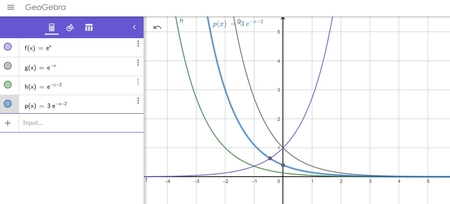# Use transformations to graph f(x) = 3e^{-x -2}

## Question:

Use transformations to graph {eq}f(x) = 3e^{-x -2} {/eq}

## Graph using Basic Transformation:

Initially we will start with the basic mother function of the form {eq}y=f(x) {/eq}.

Then by using a different type of transformations (like: Horizontal stretch, Horizontal Compression, Horizontal shifting,

Vertical stretch, Vertical Compression, Vertical shifting etc), we can easily draw the graph of the given polynomial function.

After drawing the resultant function, we can easily decide the behavior of that graph.

Mother Function: {eq}f(x)=e^x {/eq}

Now Applying transformation: "Horizontal Flip"

Function changes as: {eq}f(x)=e^{-x} {/eq}

Now Applying transformation: "Horizontal Shifting"

Function changes as: {eq}f(x)=e^{-x-2} {/eq}

Now Applying transformation: "Positive Scaling by level 3"

Function changes as: {eq}f(x)=3e^{-x-2} {/eq}

All transformations with mother function and the resultant function can be drawn as:(Graph has been drawn in geogebra.com)